# How to increase STP in IQ Bot using Custom Logic

• 209 views

Userlevel 5+9
• Automation Anywhere Team
• 7606 replies

This is a guest post from Tamil Arasu, an RPA developer with Fugro Netherlands. If you have an idea for a blog post or tutorial that you think would benefit the Automation Anywhere developer community, let us know at developer@automationanywhere.com.

In this article, we will look at how to improve/increase STP using Custom Logic with Python scripts.

First, let's understand what the Custom Logic feature in IQ Bot means.

The custom logic feature in IQ Bot enables users to make precise changes to extractions results using Python scripts. Creating automatic fine-tuning and flexible adjustments on extracted data streamlines data integration into target systems, reducing the need for human action in the process.

There are two options available to manipulate the IQ BOT output file.

The documents are uploaded to the IQ BOT learning instance and these need to be trained and validated.

During the training, we can improve the accuracy of data extraction from documents which will help to increase the straight-through processing (STP) and save time for validating documents.

Benefits: Time-saving, easy to use, avoiding repeated errors and increasing the straight-through processing day by day.

Once the documents are uploaded to the IQ BOT, we download the output files and then perform manipulations as needed and create the final output to enter the data into the ERP system or webpage or database as required

Downside: Have to create new bots to avoid repeated errors, manual entry required to validate the documents which reduce the STP when we receive new documents.

Using custom logic, we can modify data in specific fields or table extraction results.

Now, let's look at how we can perform the following operations using Custom logic:

• String manipulation
• Number format
• Date Format
• Database

### String Operations:

String manipulation is the process of changing, parsing, splicing, pasting, or analyzing strings.

Example 1: Custom logic to change the text to Uppercase and Lowercase:

Input Data: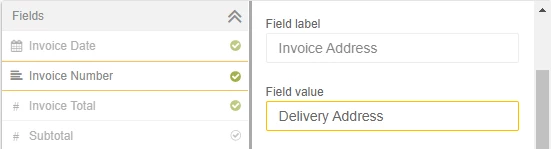Custom logic:

• Convert to Uppercase: field_value=field_value.upper()
• Convert to Lowercase:field_value=field_value.lower()

Output: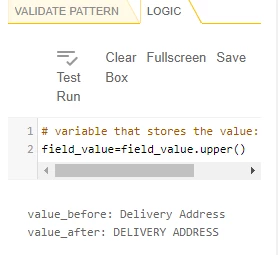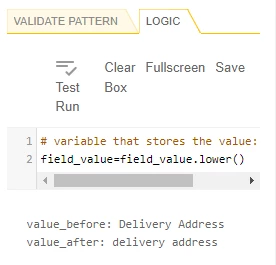Example 2: Removing unwanted whitespace and characters:

Input Data: Invoice field value has an unwanted character '/' and whitespace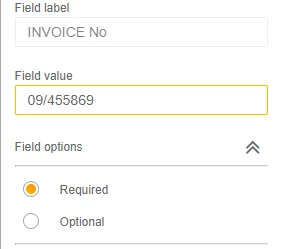Custom logic to remove the forward-slash and hidden whitespace from the field value.

• field_value = field_value.replace("/", "")
• field_value = field_value.replace(" ", "")

Output: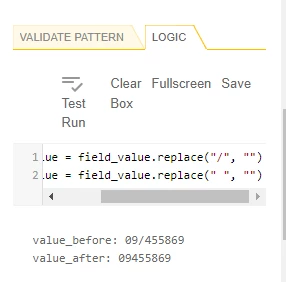Example 3: String replacement at a specific position

Sometimes, due to the document quality characters are recognized incorrectly. For example, O is displayed as 0, character as a Number and so on.

Let's look at how to replace the text at position 1.

Logic:

• string = field_value
• position = 0
• new_character = 'I'
• string = string[:position] + new_character + string[position+1:]
• field_value=string

Input & Output: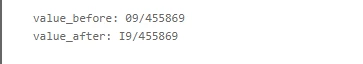Example 4: Split string

Logic:

• A = field_value.split("/")
• field_value=A

Input & Output: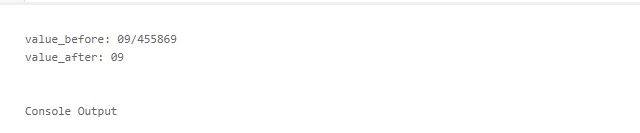### Working with Numbers

While working with Numbers, when we select the data type as the number, most of the issues get resolved, however, we will still have to face a couple of errors/issues as discussed below.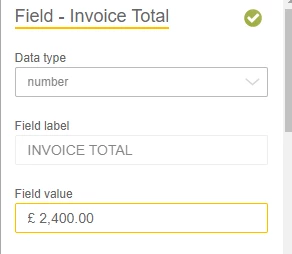Based on the value in the screenshot above, let's perform the following manipulations:

• Remove the unwanted strings from number
• Remove hidden spaces
• Remove decimal separator (,) Comma “2,400.00”
• Replace decimal separator (.) Dot with a Comma “2,400.00”

Note: Decimal separator will change based on the languages.

For removing strings from numbers, we can use String operations to remove the string and hidden/white space from the field value.

• field_value = field_value.replace("£", "")
• field_value = field_value.replace(" ", "")

Logic to Change the Decimal separator (,) Comma in “2,400.00”:

• A = len(field_value)
• B = A -3
• C = A -2
• F = A -1
• D = field_value[B:C:F]
• if D == ".":
• field_value = field_value.replace(",","").replace(".",",")

Input & Output: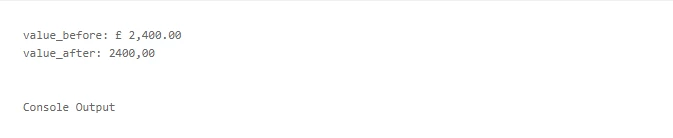Next, let's see the logic to change the Decimal separator (.) Comma in “2,400,00”

• A = len(field_value)
• B = A -3
• C = A -2
• F = A -1
• D = field_value[B:C:F]
• if D == ",":
• field_value = field_value.replace(".","").replace(",",".")

### Working with Dates

In IQ BOT, one of the major issues for documents to be redirected to the validator is due to the Date field.

Working with real-life documents, each vendor uses a different date format to share the documents and it differs from country to country.

Let's look at the logic to standardize the date format from various formats.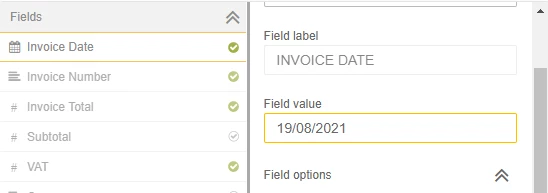Logic :

• field_value = field_value.replace("-", "")
• field_value = field_value.replace("/", "")
• field_value = field_value.replace(".", "")
• field_value = field_value.replace(",", "")
• field_value = field_value.replace(" ", "")

`import datetime `

`def format_date(field_value): `

`try: `

`#14/01/21---> 14/01/2021 `

`formated_date=datetime.datetime.strptime(field_value, '%d%m%y').strftime('%d-%m-%Y') `

`return(formated_date) `

`except ValueError: `

`try: `

`#January 20, 2021---> 20/10/2021 `

`formated_date=datetime.datetime.strptime(field_value, "%B%d%Y").strftime('%d-%m-%Y') `

`return(formated_date) `

`except : `

`try: `

`#01-Jun-21---> 01/06/2021 `

`formated_date=datetime.datetime.strptime(field_value, '%d%b%y').strftime('%d-%m-%Y') `

`return(formated_date) `

`except : `

`try: `

`#21 January 2021---> 21/01/2021 `

`formated_date=datetime.datetime.strptime(field_value, "%d%B%Y").strftime('%d-%m-%Y') `

`return(formated_date) `

`except : `

`try: `

`#21 Jan 2021---> 21/01/2021 `

`formated_date=datetime.datetime.strptime(field_value, "%d%b%Y").strftime('%d-%m-%Y') `

`return(formated_date) `

`except : `

`try: #21/01/2021---> 21/01/2021 `

`formated_date=datetime.datetime.strptime(field_value, '%d%m%Y').strftime('%d-%m-%Y') `

`return(formated_date) `

`except: `

`try: `

`#Jan 21 2021---> 21/01/2021 `

`formated_date=datetime.datetime.strptime(field_value, "%b%d%Y").strftime('%d-%m-%Y') `

`return(formated_date) `

`except: `

`try: #01/01/2021---> 01/01/2021 `

`formated_date=datetime.datetime.strptime(field_value, "%m%d%Y").strftime('%d-%m-%Y') `

`return(formated_date) `

`except: `

`return(field_value) `

`Date1=format_date(field_value) `

`field_value=Date1`

Above logic will help us to convert the date format for most of the date formats used by most suppliers/vendors.

Input & Output: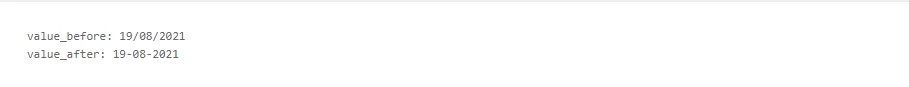### Working with Currency:

Most of the time, suppliers/vendors mention the currency value within Total amount. When we enter the data into the ERP system, we need to know the currency as well. In the example below, let's see how to identify the currency.Logic :

if "AUD" in field_value:

field_value="AUD"

elif "\$" in field_value:

field_value="USD"

elif "NZD" in field_value:

field_value="NZD"

elif "€" in field_value:

field_value="EUR"

elif "EUR" in field_value:

field_value="EUR"

elif "eur" in field_value:

field_value="EUR"

elif "USD" in field_value:

field_value="USD"

elif "£" in field_value:

field_value="GBP"

elif "Pound Sterling" in field_value:

field_value="GBP"

elif "S\$" in field_value:

field_value="SGD"

Input & Output: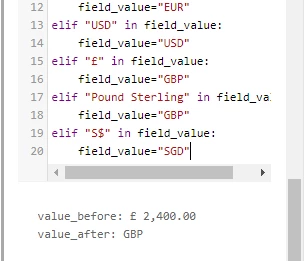### Extracting data from Tables

The table sections are always dynamic and are in different positions, especially when we receive the documents with multiple pages.

For the table section one logic tab is available for an entire table, however, to manipulate the table we have to use separate logic for each column.

In the screenshot below, let's work to remove the \$ sign from Unit price.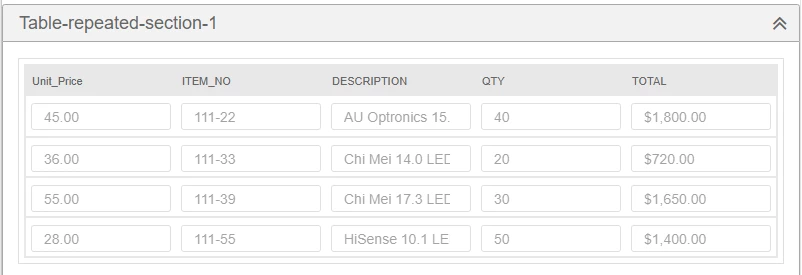Removing the \$ symbol from Unit price (if it contains \$ symbol, I know it is not present in this example but you will see this in most of the documents) and Total Columns,

import pandas as pd

import re

df = pd.DataFrame.from_dict(table_values)

df["Unit_Price"] = df["Unit_Price"].replace({'\$':''}, regex=True)

df["TOTAL"] = df["TOTAL"].replace({'\$':''}, regex=True)

table_values = df.to_dict()

Output: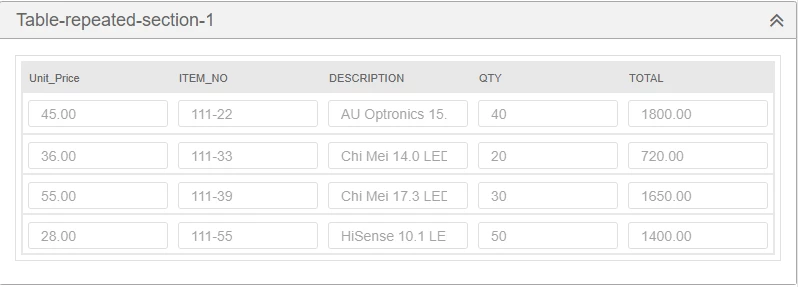Note: Changing the datatype from Text to Number also helps here, however sometimes due to the document quality, IQ BOT will capture additional characters as well.

### To remove the empty rows in Table

If the table contains with empty rows which we want to remove before downloading the output file, here is the logic:

import pandas as pd

df = pd.DataFrame.from_dict(table_values)

df = df[(df["unit_price"] != "")]

table_values = df.to_dict()

### Validating the PO/Invoice No/Order Number by connecting to Database

Organizations have their own finance team process (AP,AR..etc) document and maintain purchases and sales data etc.,

For example, organizations have their own or use Third party ERP systems. In this system, all the operational details are maintained by the Finance team.

Let's look at how to get the vendor name using Purchase order. Here is the logic for the same:

import pyodbc

conn = pyodbc.connect('Driver={SQL Server};'

'Server=localhostsqlexpress;'

'Database=purchaseorder;'

'Trusted_Connection=no;'

cursor = conn.cursor()

cursor.execute('SELECT vendor_id FROM [purchaseor-der].[dbo].[VENDOR_INFO_1] where vendor_name_short = ''+field_value+''')

for row in cursor:

field_value = row

The above query helps us to retrieve data from database which is related to the purchase order number.

### Conclusion

I hope you enjoyed and learned how to use custom logic using Python scripts to improve Straight-through processing in IQ BOT and gives you a head-start to explore what's possible to be manipulated before the output file is generated.Tamil Arasu

Tamil is a RPA Developer with Fugro Netherlands, he has 5 years of experience in automation working with multiple domains such as Telecom, Finance and Healthcare. He has hands-on experience in .NET (VB.NET, ASP.NET, C#), and experience working in Agile Methodology - Scrum Jira. He has sound technical knowledge in .Net, R Studio, Python, Power BI, Zoom Data and ELK.

When he’s not building bots, you can find Tamil on LinkedIn as well as on the forums of APeople.+1

I am starting with AA.

what’s wrong with python or AA . I am working using windows 7. I installed python 3.3.2 in my pc.